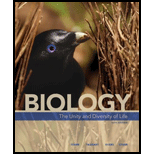# Red-flowering snapdragons are homozygous for allele R I . White-flowing snapdragons are homozygous for a different allele ( R 2 ). Heterozygous plants ( R 1 R 2 ) bear pink flowers. What phenotypes should appear among first-generation offspring of the crosses listed? What are the expected proportions for each phenotype? a. R 1 R 1 × R 1 R 2 b. R 1 R 1 × R 2 R 2 c. R 1 R 2 × R 1 R 2 d. R 1 R 2 × R 2 R 2### Biology: The Unity and Diversity o...

15th Edition
Cecie Starr + 3 others
Publisher: Cengage Learning
ISBN: 9781337408332

#### Solutions

Chapter
Section### Biology: The Unity and Diversity o...

15th Edition
Cecie Starr + 3 others
Publisher: Cengage Learning
ISBN: 9781337408332
Chapter 13, Problem 9GP
Textbook Problem
274 views

## Red-flowering snapdragons are homozygous for allele RI. White-flowing snapdragons are homozygous for a different allele (R2). Heterozygous plants (R1 R2) bear pink flowers. What phenotypes should appear among first-generation offspring of the crosses listed? What are the expected proportions for each phenotype? a. R1R1 × R1R2 b. R1R1 × R2R2 c. R1R2 × R1R2 d. R1R2 × R2R2

a.

Summary Introduction

To explain: The phenotype of the first generation offspring and expected proportion of each phenotype for R1R1 × R1R2.

Concept introduction: Incomplete dominance is a type of Non-Mendelian inheritance where the heterozygous phenotype is the intermediate of the homozygous dominant and homozygous recessive phenotypes.

### Explanation of Solution

A monohybrid cross is constructed with Punnett square for the parents having the genotype R1R1 and R1R2...

b.

Summary Introduction

To explain: The phenotype of the first generation offspring and expected proportion of each phenotype for R1R1 × R2R2.

Concept introduction: Incomplete dominance is a type of Non-Mendelian inheritance where the heterozygous phenotype is the intermediate of the homozygous dominant and homozygous recessive phenotypes.

c.

Summary Introduction

To explain: The phenotype of the first generation offspring and expected proportion of each phenotype for R1R1 × R2R2.

Concept introduction: Incomplete dominance is a type of Non-Mendelian inheritance where the heterozygous phenotype is the intermediate of the homozygous dominant and homozygous recessive phenotypes.

d.

Summary Introduction

To explain: The phenotype of the first generation offspring and expected proportion of each phenotype for R1R2× R2R2.

Concept introduction: Incomplete dominance is a type of Non-Mendelian inheritance where the heterozygous phenotype is the intermediate of the homozygous dominant and homozygous recessive phenotypes.

### Still sussing out bartleby?

Check out a sample textbook solution.

See a sample solution

#### The Solution to Your Study Problems

Bartleby provides explanations to thousands of textbook problems written by our experts, many with advanced degrees!

Get Started

Find more solutions based on key concepts
People most often choose foods for the nutrients they provide. T F

Nutrition: Concepts and Controversies - Standalone book (MindTap Course List)

What is the difference between an isotope and an ion?

Horizons: Exploring the Universe (MindTap Course List)

What is the first element of the scientific method?

An Introduction to Physical Science

A small container of water is placed on a turntable inside a microwave oven, at a radius of 12.0 cm from the ce...

Physics for Scientists and Engineers, Technology Update (No access codes included)